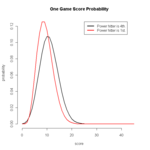# 打順を変えたときの得点```# パラメータ
library(MCMCpack)
library(gtools)
library(expm)
Nbase<- 4
Nout<- 3
Ninning<- 9
people<- 9
hitp<- 1-colMeans(team)
Vnum<- 100
cut<- 0.95
```
```# 比較してみたいチーム
Tigars<- rbind(c(0,0,0,0,1),
c(0.6508972,0.2593801,0.05709625,0.004893964,0.027732463),
c(0.6504065,0.2926829,0.04471545,0.010162602,0.002032520),
c(0.6991304,0.2034783,0.05391304,0.010434783,0.033043478),
c(0.7039007,0.1861702,0.02659574,0.000000000,0.083333333),
c(0.7592068,0.1614731,0.03399433,0.000000000,0.045325779),
c(0.6967509,0.1985560,0.05234657,0.000000000,0.052346570),
c(0.7453416,0.1614907,0.07453416,0.000000000,0.018633540),
c(0.9000000,0.1000000,0.00000000,0.000000000,0.000000000)
)
b1<- mapply(rep,2:people,1:(people-1))
b2<- mapply(rep,2:people,(people-1):1)
b<-  diag(1,people)
b[upper.tri(b)]<- unlist(b1)
b[lower.tri(b)]<- unlist(b2)
Tigars.array<- array(0,c(people,Nbase+1,2))
team.array<- array(0,c(people,Nbase+1,people))
for(t in 1:people){
team.array[,,t]<- Tigars[b[t,],]
}
```
```# プログラムに必要な自作関数
num.count<- function(vec){
counter<- rep(0,Nbase)
for(result in 1:Nbase){
counter[result]<- length(which(vec==result))
}
return(c(Nout,counter))
}

score<- function(vec){
runner<- rep(0,Nbase)
for(k in 1:length(vec)){
runner<- c(runner,c(1,rep(0,(vec[k] - 1))))
}
runner<- runner[1:(length(runner) - (Nbase - 1))]
return(sum(runner))
}

Prob<- function(vec){
P<- choose((Nout+length(vec)-1),(Nout-1))*prod((Bprob^num.count(vec)))
return(c(score(vec),P))
}

multiple.array<- function(Array){
ArrayMatrix<- Array[,,1]
for(Ar in 2:dim(Array)){
ArrayMatrix<- ArrayMatrix %*% Array[,,Ar]
}
return(ArrayMatrix)
}

h<- function(vec){
v<- rep(0,length(vec))
Ps<- which(vec!=0)
num<- vec[Ps]
v[Ps:1]<- Pn[num:(num-Ps+1)]
if(Ps!=length(vec)){
v[(Ps+1):length(v)]<- Pn[1:(length(v)-Ps)]
}else{
v<- Pn[1:length(v)]
}
return(v)
}
```
```# さあ、やろう。
data<- lapply(mapply(rep,0,1:people),unique)
for(q in 1:people){
team<- team.array[,,q]
hitp<- 1-colMeans(team)
m1<- mapply(seq,1:people,people)
m2<- mapply(seq,1,1:people)
m<- list(0)
for(i in 1:(people-1)){
m<- c(m,list(0))
}
for(y in 1:people){
m[[y]]<- unique(c(m1[[y]],m2[[y]]))
}
TB<- matrix(unlist(m),people,people,byrow=T)
team.array<- array(0,c(people,(Nbase+1),people))
for(k in 1:people){
team.array[,,k]<- team[TB[k,],]
}
A<- rep(0,Vnum)
for(v in 1:Vnum){
A[v]<- choose((v-1),(Nout-1))*(hitp)^(v-Nout)*(1-hitp)^(Nout-1)*(1-hitp)
}
Nbatter<- length(bboxesprob)
Result<- matrix(0,nr=people,nc=(Nbatter-Nout+1))
dimnames(Result)<- list(1:people,0:(ncol(Result)-1))
names(dimnames(Result))<- c("1st","Score probability")
for(ta in 1:dim(team.array)){
team<- team.array[,,ta]
if(nrow(team)<Nbatter){
for(y in 1:(Nbatter %/% nrow(team))){
team<- rbind(team,team)
}
}
for(batters in 1:(Nbatter-Nout)){
a<- p<- list(c(2:(Nbase+1)))
if(batters != 1){
for(cycles in 1:(batters-1)){
p<- c(p,a)
}
}
Bpatterns<- expand.grid(p)
cmb<- combinations(Nout+batters-1,Nout-1)
Y<- matrix(1,nrow(cmb)*nrow(Bpatterns),(Nout-1)+ncol(Bpatterns))
for(i in 1:nrow(cmb)){
for(t in 1:ncol(Bpatterns)){
Y[(1+nrow(Bpatterns)*(i-1)):((nrow(Bpatterns)*i)),
(1:ncol(Y))[-c(cmb[i,])][t]] <- Bpatterns[[t]]
}
}
SCORES<- S<- apply((Bpatterns-1),1,score)
for(i in 1:(nrow(cmb)-1)){
SCORES<- c(SCORES,S)
}
probs<- rep(1,nrow(Y))
for(NR in 1:nrow(Y)){
for(NC in 1:ncol(Y)){
probs[NR]<- probs[NR]*team[NC,Y[NR,NC]]
}
}
probs<- probs*team[ncol(Y)+1,1]
for(sc in 0:max(SCORES)){
Result[ta,sc+1]<- Result[ta,sc+1]+sum(probs[which(SCORES==sc)])
}
}
Result[ta,1]<- Result[ta,1]+prod(team[1:Nout,1])
}
Result<- Result/rowSums(Result)
#######################################################
rest<- ceiling(length(bboxesprob)/people)*people-length(bboxesprob)
bbp<- c(bboxesprob,rep(0,rest))
bp<- matrix(bbp,nr=ceiling(length(bboxesprob)/people),byrow=T)
Pn<- colSums(bp)
L<- apply(diag(people,people),2,h)
t<- c(1,rep(0,people-1))
R<- matrix(0,people,Ninning)
R[,1]<- t
for(i in 2:Ninning){
R[,i]<- L%*%R[,i-1]
}
###############################################################
I<- matrix(0,nr=Ninning,nc=ncol(Result)) # one inning score prob
for(w in 1:Ninning){
I[w,]<- colSums(Result*R[,w])
}
############################################################
M1<- array(0,
c(nr=(ncol(I)-1)*Ninning+ncol(I),
nc=(ncol(I)-1)*Ninning+1,
Ninning)
)
M <- array(0,
c(nr=(ncol(I)-1)*Ninning+1,
nc=(ncol(I)-1)*Ninning+1,
Ninning)
)
for(b in 1:Ninning){
M1[1:ncol(I),1,b]<- I[b,]
for(j in 2:ncol(M[,,b])){
M1[j:(ncol(I)+j-1),j,b]<- I[b,]
}
}
m<- M[,1,1]
GameResult<- t(multiple.array(M)%*%m)
data[[q]]<- c(GameResult)
}

xg<- max(unlist(lapply(data,length)))
yg<- max(unlist(lapply(data,max)))
xx<- 0:(xg-1)
color<- c("black","red","orange","yellow","green","blue","skyblue","purple","violet")
for(pl in 1:people){
plot(xx,data[[pl]],type="l",lwd=2,frame=F,xlim=c(0,xg),ylim=c(0,yg),col=color[pl],
xlab="score",ylab="probability",main="One Game Score Probability"
)
par(new=T)
}
axis(1:2)
legend(25,yg,c("1st","2nd","3rd","4th","5th","6th","7th","8th","9th"),
col=color,lty=1,lwd=3)
```